# Solving Equations with Fractions Worksheet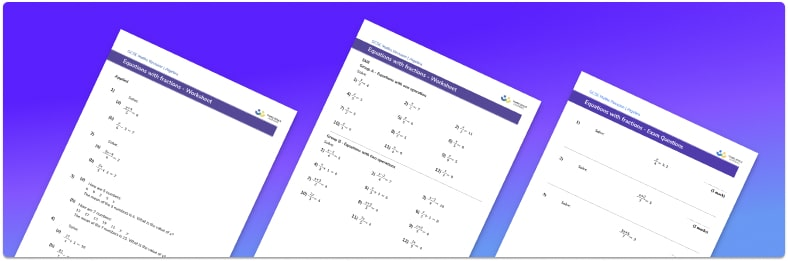• This field is for validation purposes and should be left unchanged.

You can unsubscribe at any time (each email we send will contain an easy way to unsubscribe). To find out more about how we use your data, see our privacy policy.

• Section 1 contains 36 skills-based solving equations with fractions questions, in 3 groups to support differentiation
• Section 2 contains a mix of worded problems and deeper problem solving questions
• Section 3 contains foundation and higher level GCSE exam style equations to practise solving equations with fractions
• Answers and a mark scheme are provided
• Questions follow variation theory with plenty of opportunities for students to work independently at their own level
• All questions are created by fully qualified expert secondary maths teachers
• Suitable for GCSE maths revision for AQA, OCR and Edexcel exam boards

### Solving equations with fractions at a glance

Solving equations with fractions involves equations with an algebraic expression in the numerator and/or the denominator. We can solve these equations by carefully rearranging the equation to make the unknown variable the subject by applying inverse operations. Understanding the order of operations is essential in order to get the steps required to rearrange in the correct order. The solutions to equations involving fractions can be integers (whole numbers), decimals, or fractions.

One step equations with fractions involve the unknown variable being part of the numerator of the algebraic fraction and may only require multiplying both sides of the equation by the denominator to find the solution. When the unknown variable is part of the denominator of the fraction it becomes a two-step equation or even a multi-step equation and requires more steps to solve it. The algebraic expressions in the fractions may also include parentheses (brackets) which can be expanded using the distributive property.

Skills needed for numerical fractions are very important when solving equations with fractions including finding common factors of the numerator and denominator in order to simplify it. Finding a common denominator of several fractions will enable them to be written as a single fraction, which can be simplified further by adding or subtracting the coefficients of the like terms.

Looking forward, students can then progress to additional algebra worksheets, for example a rearranging equations worksheet or a simultaneous equations worksheet.For more teaching and learning support on Algebra our GCSE maths lessons provide step by step support for all GCSE maths concepts.

## Related worksheets

Completing The Square Worksheet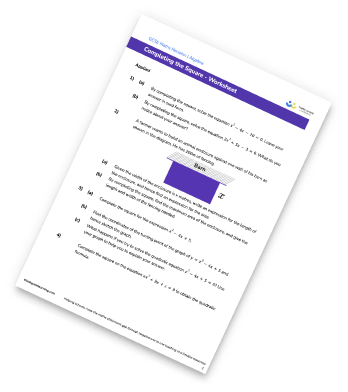Linear Equations Worksheet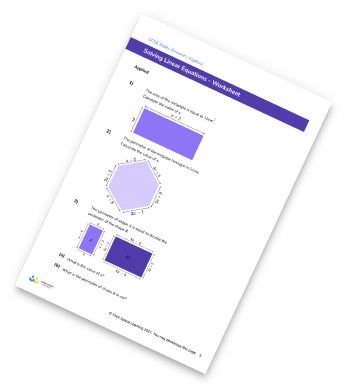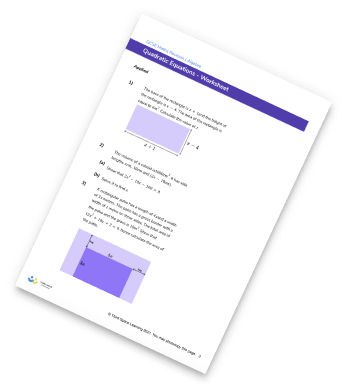Solving Equations Worksheet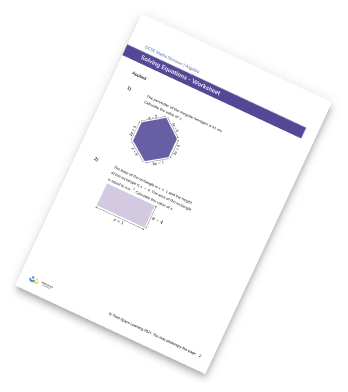## Do you have KS4 students who need more focused attention to succeed at GCSE?There will be students in your class who require individual attention to help them succeed in their maths GCSEs. In a class of 30, it’s not always easy to provide.

Help your students feel confident with exam-style questions and the strategies they’ll need to answer them correctly with our dedicated GCSE maths revision programme.

Lessons are selected to provide support where each student needs it most, and specially-trained GCSE maths tutors adapt the pitch and pace of each lesson. This ensures a personalised revision programme that raises grades and boosts confidence.

Find out more